# Frequency compensation of a two-stages class AB amplifier

#### stefanino1993

Joined Jul 27, 2019
9
The author of the book on which I'm studying explains in depth the following "standard" two-stages op-amp: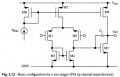In order to deal with frequency compensation, he models each stage with a transconductance amplifier: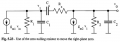in which gm1 and gm2 are the transconductances of the first and second stage, R1 and R2 are the output resistances of the first and second stage, C1 and C2 are the output parasitic capacitances of the first and second stage, respectively. Cc and Rz are introduced for the frequency compensation. In order to achieve frequency compensation, Cc must be chosen sufficiently large to get pole-splitting while Rz (which is the zero-nulling resistor) must be chosen equal to the inverse of the transconductance of the second stage.

Now I'm studying the following two-stages class AB amplifier: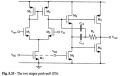Basically it is a standard two-stages op-amp to which the source follower M8-M9 has been added in order to provide the single-ended output signal of the differential pair to the gates of both M5 and M6. The author of my book says: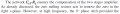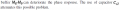1st question

It seems that the author of my book generalizes the small-signal equivalent circuit of figure 5.23 also to the class-ab two-stages op-amp, ignoring the source follower stage M8-M9 (I imagine because it has more or less a unity gain). Is my assumption right? If this is the case, then I surmise that the zero-nulling resistor Rz must be equal to 1/(gm5+gm6) which is the inverse of the transconductance of the second stage M5-M6

2nd question

The author only says that, since the source follower M8-M9 has a given frequency response, then the capacitor Cc2 "attenuates" possible problems, but he does not explain two things:

• why is capacitor Cc2 placed right there?
• with which criterion should I choose its value?